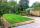# Plot

The length of the rectangle is 8 smaller than three times the width. If we increase the width by 5% of the length and the length is reduced by 14% of the width, the circumference of rectangle will be increased by 30 m. What are the dimensions of the rectangle?

Result

a =  4612 m
b =  1540 m

#### Solution:

a = 3b-8
2(1.05a + (1-0.14)*b) = 30 + 2*(a+b)

a-3b = -8
0.1a-0.28b = 30

a = 4612
b = 1540

Calculated by our linear equations calculator.

Leave us a comment of this math problem and its solution (i.e. if it is still somewhat unclear...):Be the first to comment!#### Following knowledge from mathematics are needed to solve this word math problem:

Our percentage calculator will help you quickly calculate various typical tasks with percentages. Do you have a system of equations and looking for calculator system of linear equations? Do you want to convert length units?

## Next similar math problems:

1. PropertyThe length of the rectangle-shaped property is 8 meters less than three times of the width. If we increase the width 5% of a length and lendth reduce by 14% of the width it will increase the property perimeter by 13 meters. How much will the property cost
2. ParallelogramThe perimeter of the parallelogram is 417 cm. The length of one side is 1.7-times longer than the length of the shorter side. What is the length of sides of a parallelogram?
3. RectangleThe width of the rectangle is 65% of its length. Perimeter of the rectangle is 132 cm. Determine the dimensions of the rectangle.
4. Rectangle SSPerimeter of a rectangle is 268 cm and its diagonal is 99.3 cm. Determine the dimensions of the rectangle.
5. Triangle - is RT?Triangle has a circumference of 90 cm. Side b is 1 cm longer than c, side c is 31 cm longer than side a. Calculate the length of sides and determine whether triangle is a right triangle.
6. Triangle ABCIn a triangle ABC side b measure 10 cm less than the side a and side b is half of the side c. Calculate the length of sides if the circumference of the triangle is 42 cm.
7. Circle arcCircle segment has a circumference of 135.26 dm and 2096.58 dm2 area. Calculate the radius of the circle and size of central angle.
8. Isosceles triangleThe perimeter of an isosceles triangle is 112 cm. The length of the arm to the length of the base is at ratio 5:6. Find the triangle area.
9. RT leg and perimeterCalculate the length of the sides of a right triangle ABC with hypotenuse c when the length of a leg a= 84 and perimeter of the triangle o = 269.
10. Rectangle from stringString 12m. Make rectangle when one side is two times longer than its width.
11. GardensThe garden has the square shape with circumference 124 m. Divide it into two rectangular gardens, one should circumference 10 meters more than the second. What size will have a gardens?
12. RectanglePerimeter of rectangle is 48 cm. Calculate its dimensions if they are in the ratio 5:3 (width:height)
13. Rectangle - sides ratioCalculate area of rectangle whose sides are in ratio 3:13 and perimeter is 673.
14. GardenThe garden has a rectangular shape and has a circumference of 130 m and area 800.25 m2. Calculate the dimensions of the garden.
15. Right triangle AlefThe obvod of a right triangle is 84 cm, the hypotenuse is 37 cm long. Determine the lengths of the legs.
16. ParallelogramParallelogram has sides lengths in the ratio 3: 4 and perimeter 2.8 meters. Determine the lengths of the sides.
17. Two squaresTwo squares whose sides are in the ratio 5:2 have sum of its perimeters 73 cm. Calculate the sum of area this two squares.